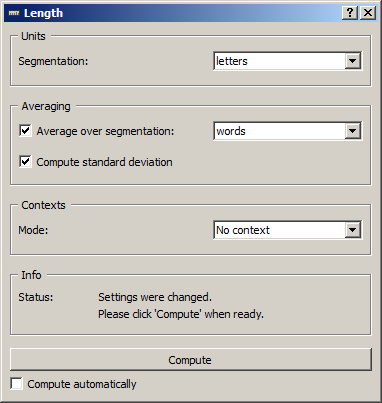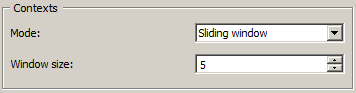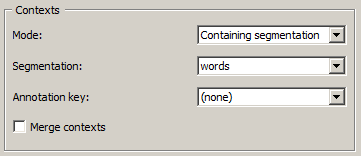# Length¶Compute the (average) length of segments.

## Signals¶

Inputs:

• `Segmentation` (multiple)

Segmentation whose segments constitute the units of length measurement, the contexts whose lengths will be measured, or the units over which length will be averaged

Outputs:

• `Textable table`

Table in the internal format of Orange Textable

## Description¶

This widget inputs one or several segmentation, measures the length of one (eventually within the segments defined by another segmentation), and sends the results in table format. It also allows the user to calculate the average length of segments of a segmentation based on the units defined by another segmentation.

The tables produced by the Length widget have at least 2 columns, and at most 4. The first column contains the headers corresponding to the contexts – which are essentially defined in the same way as in the Count widget. The second column gives the length indications (in which case the header is __length__) or the average length (header __length_average__). In the latter case, the third column may then contain the corresponding standard deviations if their display is required by the user (header __length_std_deviation__), and the last column will indicate the number of elements on which the average calculation is done (header __length_count__).

To take a simple example, consider two segmentations of the string a simple example :

1. label = words
content start end part of speech word category
a 1 1 article grammatical
example 10 16 noun lexical
1. label = letters (extract)
content start end letter category
a 1 1 vowel
s 3 3 consonant
i 4 4 vowel
... ... ... ...
e 16 16 vowel

Essentially here two basic configurations are considered. The first is when we are simply interested in the length of a given segmentation, for example letters:

__context__ __length__
__global__ 14

In what follows, we will designate with the terms units of measurement the segments whose count is interpreted as a length measure, namely in this example the segments of the segmentation letters.

The second basic configuration is when we wish to know the average length of the segments of a segmentation, for example words, in terms of measure units belonging to another segmentation (here letters):

__context__ __length_average__ __length_std_deviation__ __length_count__
__global__ 4.66666650772 2.62466931343 3

In this case, we will name averaging units the segments whose lengths are measured and averaged. Note that the average length calculation presupposes that at least one measure unit is contained within the averaging unit, in the sense that the following three conditions are met: (a) the segment corresponding to the unit and the context are both be associated to the same string, (b) the initial position of the unit segment in the string is higher or equal to that of the context segment, and (c) conversely the final position of the unit is lower or equal to that of the context.

These two elementary configurations (length measurement and average length calculation) can then be combined with two ways of specifying contexts – i.e. two ways of defining table rows. The first mode consists of defining the contexts based on the content or the annotations of a given segmentation; for example, here is the length of the words segments (contexts) in terms of those of letters (units of measurement):

__context__ __length__
a 1
simple 6
example 7

It should be noted that the segment types define the row headers, as illustrated in the following example, where the same segmentations are used but the contexts are defined by the annotation values associated with the key word type:

__context__ __length__
grammatical 1
lexical 13

The average length calculation is also applicable when the contexts are defined on the basis of a segmentation. In this case, we will generally use three different segmentations to define the units of measurement, the averaging units, and the contexts; for example, it could be to calculate the average length of words (in number of letters) in different texts. To stay in the frame of our example based on only two segmentations, we can exploit the fact that all segments are contained in themselves and calculate the average length of words (in number of letters) depending on the word types annotations (in other words we here use a single segmentation to determine the contexts and the averaging units):

__context__ __length_average__ __length_std_deviation__ __length_count__
grammatical 1 0 1
lexical 6.5 0.5 2

The second context specification mode lies on the concept of a “window” of n segments that we progressively slide from the beginning to the end of the segmentation. For example, by setting the window size to 2 segments, we can examine the average length of words (in number of letters) in successive bigrams of the words segmentation (identified by their position):

__context__ __length_average__ __length_std_deviation__ __length_count__
1 3.5 2.5 2
2 6.5 0.5 2

By construction, each cell of the column __length_count__ will then contain the same value, or the window size. Based on this observation, it is rather easy to convince oneself that this latter context specification mode only makes sense when we are interested in the evolution of an average length throughout a segmentation.

We now move on to the presentation of the widget interface (see figure 1). It contains three separate sections for the specification of the units of measurement (Units), of the averaging units (Averaging), and of the contexts (Contexts).

The Units section only contains a single drop-down menu (Segmentation) used to select among the input segmentation the one whose segments will provide the units of measurement.

In the Averaging section, the Average over segmentation checkbox triggers the calculation of the average length. The drop-down menu on the right allows the user to select the segmentation whose segments will constitute the averaging units. The Compute standard deviation checkbox allows the user to calculate, other than the average length, its standard deviation. It should be noted that for large segmentations, this option is likely to spectacularly extend the calculation time.Figure 1: Length widget (No context mode).

The Contexts section is available in several variants depending on the value selected in the Mode drop-down menu. This latter option allows the user to choose among the context specification modes described above. The No context mode corresponds to the case where the length measurement or the average length calculation are globally applied to the entire segmentation that defines the units of measurement (specified in the Units section).

The Sliding window mode (figure 2) implements the notion of a “sliding window” introduced above. It allows the user to observe the evolution of the average length throughout the averaging unit segmentation. The only parameter is the size of the window (in number of segments), set by means of the Window size cursor.Figure 2: Length widget (Sliding window mode).Figure 3: Length widget (Containing segmentation mode).

Finally, the Containing segmentation mode (see figure 3) corresponds to the case where the contexts are defined by the segment types appearing in a segmentation (that will most often be distinct from the segmentation providing the units of measurement and the averaging units). This segmentation is selected among the input segmentation by means of the Segmentation drop-down menu. The Annotation key menu shows the possible annotation keys associated to the selected segmentation; if one of these keys is selected, the corresponding types of annotation values will constitute the row headers; if on the other hand the value (none) is selected, the content of the segments will be used. The Merge contexts checkbox allows the user to measure the length or to calculate the average length globally in the entire segmentation that defined the contexts.

The Info section indicates if a table has been correctly emitted, or the reasons why no table is emitted (no input data).

The Compute button triggers the emission of a table in the internal format of Orange Textable to the output connection(s). When it is selected, the Compute automatically checkbox disables the button and the widget attempts to automatically emit a segmentation at every modification of its interface or when its input data are modified (by deletion or addition of a connection, or because modified data is received through an existing connection).

## Messages¶

### Information¶

Data correctly sent to output.
This confirms that the widget has operated properly.
Settings and/or input have changed but the Compute automatically checkbox has not been selected, so the user is prompted to click the Compute button (or equivalently check the box) in order for computation and data emission to proceed.
No data sent to output yet: no input segmentation.
The widget instance is not able to emit data to output because it receives none on its input channel(s).
No data sent to output yet, see ‘Widget state’ below.
A problem with the instance’s parameters and/or input data prevents it from operating properly, and additional diagnostic information can be found in the Widget state box at the bottom of the instance’s interface (see Warnings below).

### Warnings¶

Resulting table is empty.
No table has been emitted because the widget instance couldn’t find a single element in its input segmentation(s). A likely cause for this problem (when using the Containing segmentation mode) is that the unit and context segmentations do not refer to the same strings, so that the units are in effect not contained in the contexts. This is typically a consequence of the improper use of widgets Preprocess and/or Recode (see Caveat).

## Footnotes¶

  By convention, we do not indicate here the string index associated with each segment but only its start and end positions, along with the various annotation values associated with it; moreover, for the sake of readability, we do indicate the content of each segment, though it is not formally part of the segmentation (but rather of the string to which the segmentation refers).